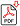Search for Articles:

## Enhancement of Heat Transfer Coefficient in DPDGSAH having V Ribs with Symmetrical Numbers of Gaps in a Rectangular Duct: A Robust Design Approach

Jolly Lucas, Pooja Tiwari

Abstract: Enhancement of heat transfer coefficient has been carried out through a solar air heater (DPDGSAH) having artificially roughened V-type geometry with symmetrical gaps and then optimized by use of Robust design method. A L25 orthogonal array is used to optimize the geometry factors for the maximum thermal performance of the ribbed channel. Thermal performance concept includes maximization of heat transfer coefficient and its thermal efficiency. Maximization of heat transfer coefficient is taken as the criteria of optimization. The investigation encompassed Reynolds number (Re) ranging from 4000 to 12000, relative roughness pitch (P/e) values of 4?12, angle of attack (?) range of 300?900, relative gap width (g/e) values of 1?5, Number of gaps (Ng) values of 1?5, and relative roughness height (e/D) values of 0.043. Results show that Reynolds Number (Re), relative roughness pitch (P/e), angle of attack (?) have the greatest influences parameters on maximization of heat transfer coefficient.

Keywords: V-Rib with symmetrical gaps, Taguchi method, ANOVA, Heat transfer coefficient.

Country: India, Subject Area: Mechanical Engineering

Pages: 328 - 335

Edition: Volume 8 Issue 3, March 2019